# 最简单的人工神经网络

08/25 08:47## 导言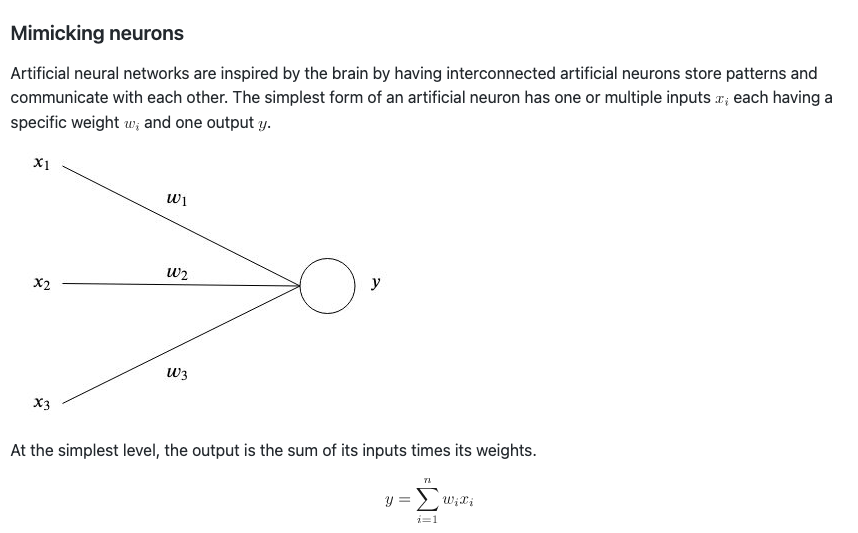• 第一部分：最简单的人工神经网络
• 第二部分：最基础的反向传播算法

温馨提示：公式虽多但只是看起来唬人，实际耐下心读并不难懂。下面正文开始！

# 一、最简单的人工神经网络

## 理论

### 模拟神经元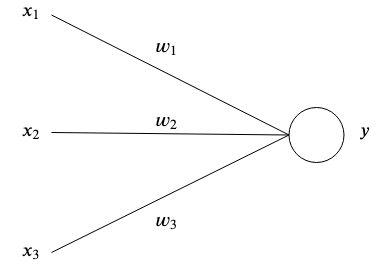y=i=1nwixi

### 一个简单的例子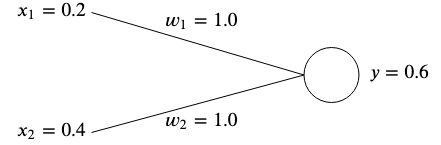y=x1w1+x2w2=0.21+0.41=0.6

### 误差值

yt=0.60.5=0.1

E=21(yt)2

E=2n1(yiti)2

y=t=0.20.5+0.41.0=0.5

### 梯度下降

#### 什么是梯度？

f(x,y)=[fx,fy]=[ðxðf(x,y),ðyðf(x,y)]

f(x,y)=[4x,9y2]

#### 什么是梯度下降？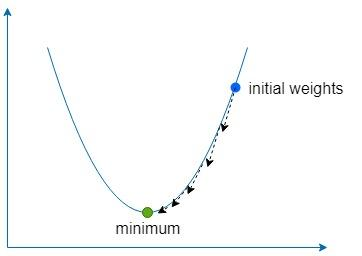wE=<ðw1ðE,ðw2ðE>

ðwiðE=ðyðEðwiðy=ðyð(21(yt)2)ðwið(xiwi)=(yt)xi

w1=w1εw1E=w1εδx1
w2=w2εw2E=w2εδx2

## 代码示例

x=[1.01.01.00.0]y=[0.01.0]

### 怎么运行？

#### Go

PS D:\github\ai-simplest-network-master\src> go build -o bin/test.exe
PS D:\github\ai-simplest-network-master\bin> ./test.exe

err:  1.7930306267024234
err:  1.1763080417089242
……
err:  0.00011642621631266815
err:  0.00010770190838306002
err:  9.963134967988221e-05
Finished after 111 iterations

Results ----------------------
[1 1] => [0.007421243532258703]
[1 0] => [0.9879921757260246]



#### Docker

docker build -t simplest-network .
docker run --rm simplest-network


# 二、最基础的反向传播算法

## 理论

### 感知机介绍

xj=i=1Ixiwij+bi

xj=i=1I+1xiwij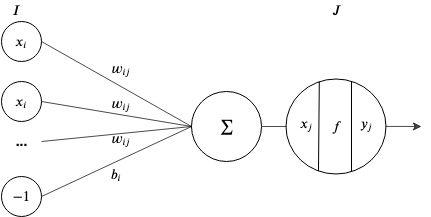### 激活函数

Sigmoid 函数 : y=1+ex1

tanh 函数： y=tanh(x)

### 反向传播

#### 术语

• xi,xj,xk​ 分别是 I, J, K 层节点的输入值。
• yi,yj,yk 分别是 I, J, K 层节点的输出值。
• yk 是 K 输出节点的期望输出值。
• wij,wjk 分别是 I 到 J 层和 J 到 K 层的权重值。
• t 代表 T 组关联中当前的一组关联。

• 输入层 -> 恒等函数
• 隐藏层 -> Sigmoid 函数
• 输出层 -> 恒等函数

#### The forward pass

xj=i=1Iwijyi

yj=fj(xj)=1+exj1

xk=j=1Jwjkyj

yk=fk(xk)=xk

E=i=1IEt=2T1t=1T(yktykt)2

#### The backward pass

Δwjk=εwjkE

wjkE=xkEwjkxk
xkE=ykExkyk=yk(21(ykyk)2)xk(xk)=ykyk=δk
wjkxk=wjk(yjwjk)=yj

Δwij=εwijE
wijE=xjEwijxj=δjyi

#### δj​计算

xjE=yjEdxjdyj
yjE=k=1KxkEyjxk=k=1Kδkwjk

dxjdyj=f(xj)=f(xj)(1f(xj))=yj(1yj)

δj=yj(1yj)k=1Kδkwjk

### 算法总结

• 对每次关联，通过神经网络向前传输，得到输出值
• 计算每个输出节点的误差 （δk=ykyk​）
• 叠加计算每个输出权重的梯度 （wjkE=δkyj
• 计算隐藏层每个节点的 δ δj=yj(1yj)k=1Kδkwjk
• 叠加计算每个隐藏层权重的梯度 （wijE=δjyj​​）
• 更新所有权重值，重置叠加梯度 （w=wεE

### 图解反向传播

#### Forward pass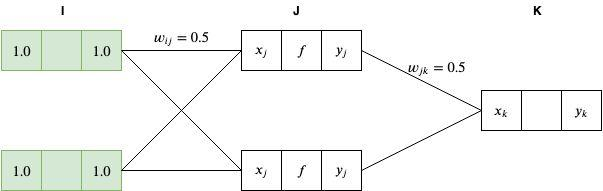xj=i=1Iyiwij=1.00.5+1.00.5=1.0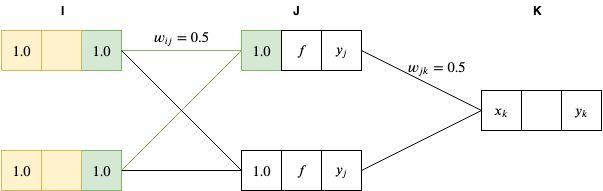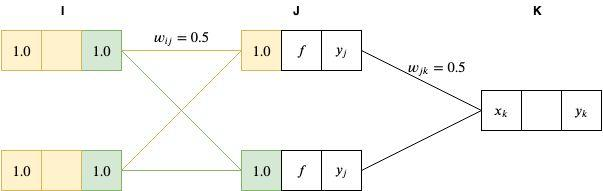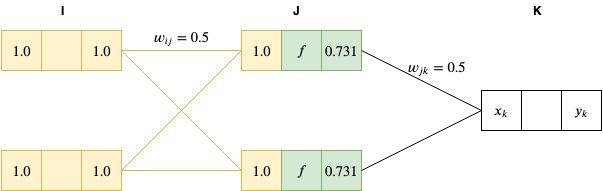xk=0.7310.5+0.7310.5=0.731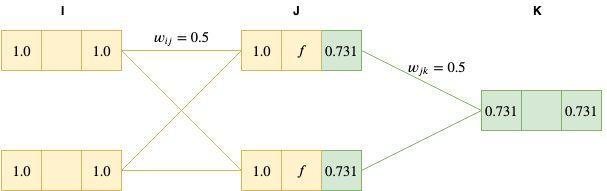#### Backward pass

δk=ykyk=0.7310.5=0.231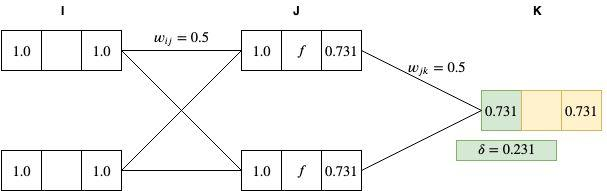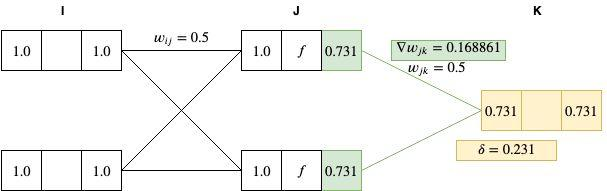δj=yj(1yj)k=1Kδkwjk=0.731(10.731)(0.50.231)0.0227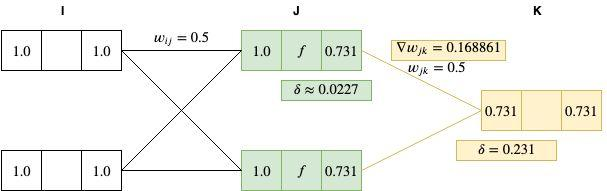wijE=δjyi=0.02271=0.0227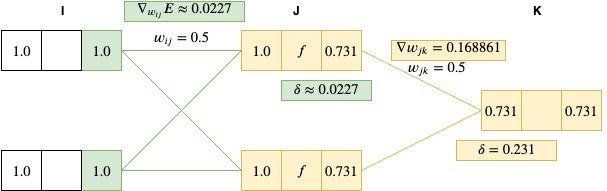wij=wijεwijE=0.50.010.0227=0.499773
wjk=wjkεwjkE=0.50.010.168861=0.49831139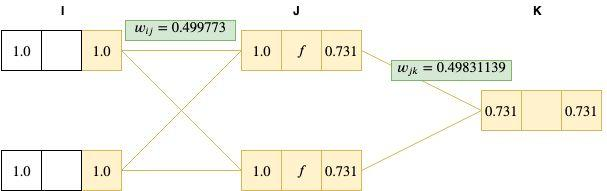## 代码示例

x=[1.01.01.00.00.01.00.00.0]
y=[0.01.01.00.0]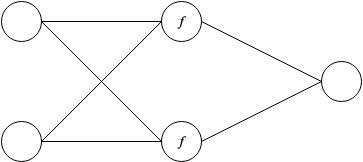## 最后

5
7 收藏

### 作者的其它热门文章0 评论
7 收藏
5